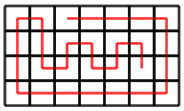CSES - Grid Path Construction
• Time limit: 1.00 s
• Memory limit: 512 MB
Given an $n \times m$ grid and two squares $a=(y_1,x_1)$ and $b=(y_2,x_2)$, create a path from $a$ to $b$ that visits each square exactly once.

For example, here is a path from $a=(1,3)$ to $b=(3,6)$ in a $4 \times 7$ grid:Input

The first input line has an integer $t$: the number of tests.

After this, there are $t$ lines that describe the tests. Each line has six integers $n$, $m$, $y_1$, $x_1$, $y_2$ and $x_2$.

In all tests $1 \le y_1,y_2 \le n$ ja $1 \le x_1,x_2 \le m$. In addition, $y_1 \neq y_2$ or $x_1 \neq x_2$.

Output

Print YES, if it is possible to construct a path, and NO otherwise.

If there is a path, also print its description which consists of characters U (up), D (down), L (left) ja R (right). If there are several paths, you can print any of them.

Constraints
• $1 \le t \le 100$
• $1 \le n \le 50$
• $1 \le m \le 50$
Example

Input:
5 1 3 1 1 1 3 1 3 1 2 1 3 2 2 1 1 2 2 2 2 1 1 2 1 4 7 1 3 3 6

Output:
YES RR NO NO YES RDL YES RRRRDDDLLLLLLUUURDDRURDRURD